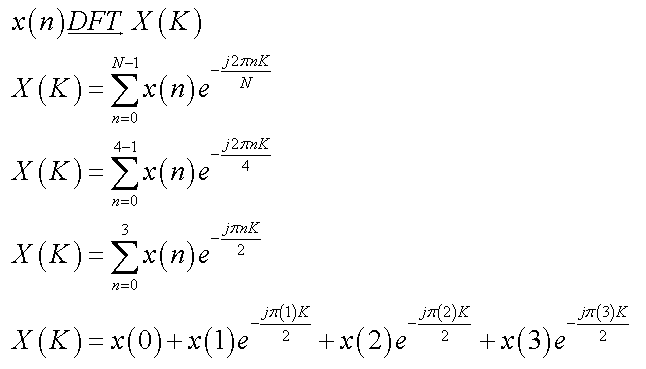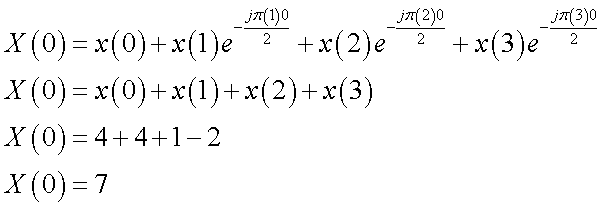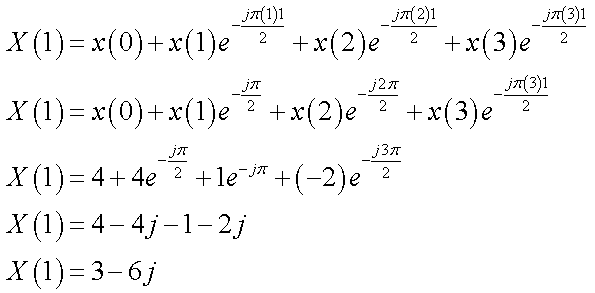# Required informationConsider the following sequence of data(x, x, x, x) = (4,4,1,–2)Without using a computer, find the forward DFT of the given sequence of data.The values of the components of the forward DFT are XD. x[1) =(Click to select) . X[2) =[). and X = (Cick to select)|

Question
2 viewshelp_outlineImage TranscriptioncloseRequired information Consider the following sequence of data (x, x, x, x) = (4,4,1,–2) Without using a computer, find the forward DFT of the given sequence of data. The values of the components of the forward DFT are X D. x[1) = (Click to select) . X[2) =[ ). and X = (Cick to select)| fullscreen
check_circle

Step 1

From the given data, the length of the sequence is 4.

Since, the DFT for the x(n) is expressed as:Step 2

Substituting the known values in the above equation.

Hence, the DFT at the desired value can be evaluated using the above expression.

At, K=0:Step 3

Similarly,

At, K=1:...

### Want to see the full answer?

See Solution

#### Want to see this answer and more?

Solutions are written by subject experts who are available 24/7. Questions are typically answered within 1 hour.*

See Solution
*Response times may vary by subject and question.
Tagged in